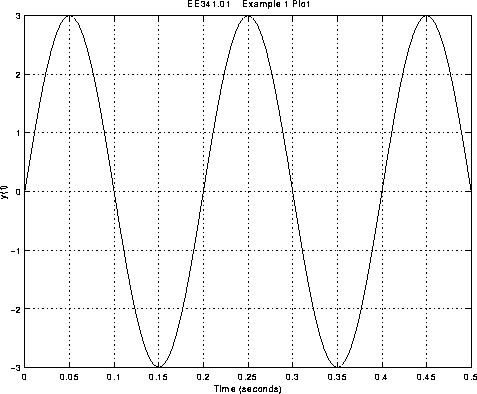#### EE341.01: MATLAB M-FILE FOR PLOTTING A SINE WAVEFORM

This example shows how a MATLAB M-file can be used to plot the sine wave of Example 1.

MATLAB M-File example2.m:
```
% File: example2.m
%
% Description:  This M-file plots a sine wave with a frequency
%               of 5Hz.
%

diary e2_diary                      % Start diary file e2_diary
amp = 3;                            % Define the amplitude
freq_HZ = 5;                        % Define the frequency in Hertz
freq_RPS = 2*pi*freq_HZ;            % Compute the frequency in rad/sec
t = 0:0.005:0.5;                    % Define the time vector
y = amp * sin(freq_RPS * t);        % Compute y(t), the sine wave
plot(t,y)                           % Plot y vs. t
xlabel('Time (seconds)')            % Label x-axis
ylabel('y(t)')                      % Label y-axis
title('EE341.01    Example 1 Plot') % Give plot a title
grid                                % Turn on plot grid
diary off                           % Turn diary off

Run example2.m by Typing example2 at the Prompt:

>>example2

Resulting e2_diary Diary File:

Note that the diary file e2_diary is empty in this case.  This
is due to no commands being typed at the prompt as well as all
the MATLAB responses being surpressed with semicolons.

MATLAB Plot Generated:The plot was printed by typing 'print' at the MATLAB
prompt when the plot was displayed in the graphics window.

```